NCERT Solutions: Rational Numbers- 2

# NCERT Solutions: Rational Numbers- 2 - Notes | Study Mathematics (Maths) Class 8 - Class 8

 1 Crore+ students have signed up on EduRev. Have you?

What is a Rational Number?

A rational number, in Mathematics, can be defined as any number which can be represented in the form of p/q where q ≠ 0.
• Also, we can say that any fraction fits under the category of rational numbers, where the denominator and numerator are integers and the denominator is not equal to zero.
• When the rational number (i.e., fraction) is divided, the result will be in decimal form, which may be either terminating decimal or repeating decimal.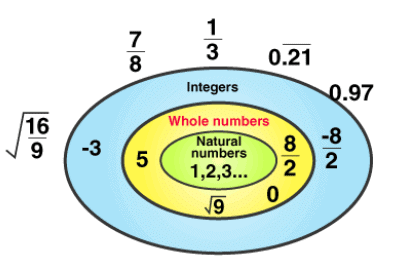Let's have a look at NCERT Solutions of Rational Numbers!

Try These

Q1. Write the rational number for each point labelled with a letter.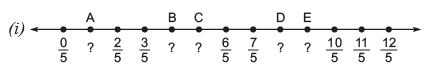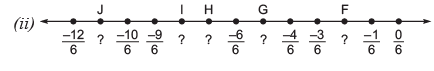Ans:
(i) Here, the rational number for the point A is 1/5
The rational number for the point B is 4/5
The rational number for the point C is 5/5 or 1
The rational number for the point D is 8/5
The rational number for the point E is 9/5

(ii) The rational number for:
The point is F -2/6 or 1/3
The point G is -5/6
The point H is -7/6
The point I is -8/6 or -43
The point J is -11/6

Exercise 1.2

Q.1. Represent these numbers on the number line.
(i) 7/4
(ii) - 5/6
Difficulty level: Medium

Reasoning: The positive numbers are on the right of 0 and negative numbers are represented on the left of 0 in the number line. The denominator of the rational number indicates the number of equal parts into which the first unit has to be divided whereas the numerator indicates as to how many of these parts are to be taken into consideration.

Solution:
7/4 The first unit has to be divided by 4 parts. We make 7 markings of distance
1/4 Each on the right of 0 and starting from 0. The seventh marking represents 7/4

Diagram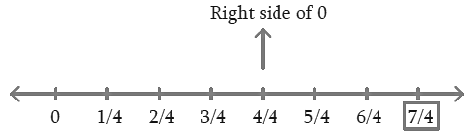-5/6 The first unit has to be divided into 6 parts. We make 5 markings of distance 1/6 each on the left of 0 and starting from 0.
The fifth marking represents 5/6 left side of 0.

Diagram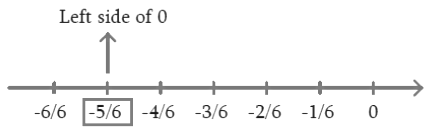Q2. Represent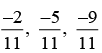on the number line.

Difficulty level: Medium

Reasoning: The negative numbers are represented on the left of 0 in the number line. The denominator of the rational number indicates the number of equal parts into which the first unit has to be divided whereas the numerator indicates as to how many of these parts are to be taken into consideration.

Solution:

We make 9 marking of distance 1/11 each on the left of 0 and starting from 0.

The second marking represent - 2/11 which is represented as A in the line.

The fifth marking represent -5/11 representations B in the line.

The ninth marking represent -9/11 represented as C in the number line.

Diagram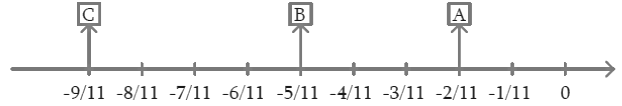Q.3. Write five rational numbers which are smaller than 2.
Difficulty level: Easy
What is the known/given?

Rational numbers.

What is the unknown?

The rational numbers between given rational numbers.

Reasoning: We can write infinitely many rational numbers lesser than number 2.

Solution:

Five rational numbers which are smaller than 2 are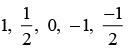Q.4.  Find ten rational numbers between -2/5 and 1/2
Difficulty level: Medium

What is the known/given?

Rational numbers.

What is the unknown?

The rational numbers between given rational numbers.

Reasoning:

We can find infinitely many rational numbers between any two given rational numbers by taking the mean of the two rational numbers. Another method: We can make the denominator same for the two given rational numbers.

Solution: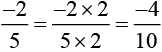[multiplying both numerator and denominator by 2]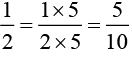[multiplying both numerator and denominator by 5]

[Multiplying both numerators and denominators by the same number]
Now, there are 9 rational numbers between -4/10 and 5/10, but we need 10 numbers. So, we should again multiply both numerator and denominator by 2 in the two rational numbers -4/10 and 5/10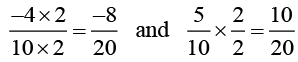The ten rational numbers between -2/5 and 1/2 which can be taken as.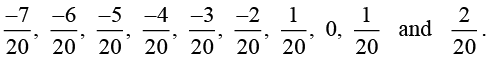[There are many more such rational numbers.]

Q.5. Find five rational numbers between,

(i) 2/3 and 4/5
(ii) -3/2 and 5/3
(iii) 1/4 and 1/2
Difficulty level: Medium
What is the known/given?

Rational numbers.

What is the unknown?

The rational numbers between given rational numbers.

Reasoning: We can find infinitely many rational numbers between any two given rational numbers by taking the mean of the two rational numbers.
Another method: We can make the denominator same for the two given rational numbers.

Solution:

(i) 2/3 and 4/5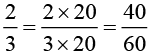[multiplying both numerator and denominator by 20]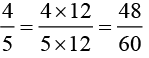[multiplying both numerator and denominator by 12]

Answer: The five rational numbers between 2/3 and 4/5 that can be taken are: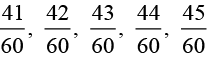Solution:
(ii)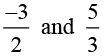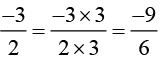[multiplying both numerator and denominator by 3]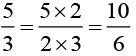[multiplying both numerator denominator by 2]

∴ The five rational numbers between -3/2 and 5/3 that can be taken are: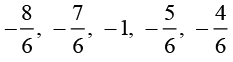[There can be more such rational numbers]

(iii) Solution:

1/4 and 1/2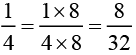[multiplying both numerator and denominator by 8]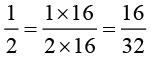[multiplying both numerator and denominator by 16]

Thus, five rational numbers between 1/2 and 1/4 that can be taken are: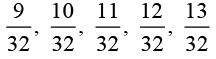Q.6. Write five rational numbers greater than –2.

Difficulty level: Medium

What is the known/given?

Rational numbers.

What is the unknown?

The rational numbers between given rational numbers.

Reasoning: We can find infinitely many rational numbers between any two given rational numbers by taking the mean of the two rational numbers. Another method: We can make the denominator same for the two given rational numbers.

Solution:

Some of the five rational numbers greater than – 2 are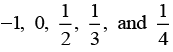[There can be more such rational numbers]

Q.7. Find the rational numbers between 3/5 and 3/4
Difficulty level: Medium

What is the known/given?

Rational numbers.

What is the unknown?

The rational numbers between given rational numbers.

Reasoning: We can find infinitely many rational numbers between any two given rational numbers by taking the mean of the two rational numbers. Another method: We can make the denominator same for the two given rational numbers.

Solution: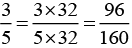[multiplying both numerator and denominator by 32]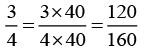[multiplying both numerator and denominator by 40]

Thus, ten rational numbers between 3/5 and 3/4 that can be written are: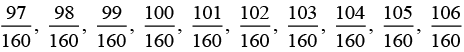[There can be many more such rational numbers]

The document NCERT Solutions: Rational Numbers- 2 - Notes | Study Mathematics (Maths) Class 8 - Class 8 is a part of the Class 8 Course Mathematics (Maths) Class 8.
All you need of Class 8 at this link: Class 8

## Mathematics (Maths) Class 8

193 videos|360 docs|48 tests
 Use Code STAYHOME200 and get INR 200 additional OFF

## Mathematics (Maths) Class 8

193 videos|360 docs|48 tests

Track your progress, build streaks, highlight & save important lessons and more!

,

,

,

,

,

,

,

,

,

,

,

,

,

,

,

,

,

,

,

,

,

;